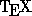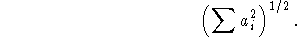Next: Matrices Up: Commands for Math Mode Previous: Getting Stuff On Top

## Bracketing

You can get all different kinds of sizes of parentheses, brackets, curly brackets, etc. to make your formulas look good. Typing

$$\Biggl( \biggl( \Bigl( \bigl( ( ) \bigr) \Bigr) \biggr) \Biggr)$$
gives youAnalogous commands work for [ and \{. Note theused to get curly braces.

You can also letfigure out how big a pair of parentheses should be to fit the contained expression. Use \left( and \right), like this:

$$\left(\sum a_i^2\right)^{1/2}$$
givesOf course, you can also use \left[, \right], \left\{ and \right\}.

Processed by LaTeX2html